Solving unsteady Schrödinger equation using the improved element-free Galerkin method
Faculty of Maritime and Transportation, Ningbo University, Ningbo 315211, China
Shanghai Institute of Applied Mathematics and Mechanics, Shanghai University, Shanghai 200072, China

† Corresponding author. E-mail: ymcheng@shu.edu.cn

Project supported by the National Natural Science Foundation of China (Grant No. 11171208), the Natural Science Foundation of Zhejiang Province, China (Grant No. LY15A020007), the Natural Science Foundation of Ningbo City (Grant No. 2014A610028), and the K. C. Wong Magna Fund in Ningbo University, China.

Abstract
Abstract

By employing the improved moving least-square (IMLS) approximation, the improved element-free Galerkin (IEFG) method is presented for the unsteady Schrödinger equation. In the IEFG method, the two-dimensional (2D) trial function is approximated by the IMLS approximation, the variation method is used to obtain the discrete equations, and the essential boundary conditions are imposed by the penalty method. Because the number of coefficients in the IMLS approximation is less than in the moving least-square (MLS) approximation, fewer nodes are needed in the entire domain when the IMLS approximation is used than when the MLS approximation is adopted. Then the IEFG method has high computational efficiency and accuracy. Several numerical examples are given to verify the accuracy and efficiency of the IEFG method in this paper.

1. Introduction

As a fundamental model to describe the nonlinear phenomenon of nature, the nonlinear Schrödinger (NLS) equation is widely used in kinds of fields, such as nonlinear optical medium, photonics, plasmas, Bose–Einstein condensates,[6,7] quantum mechanics, electro-magnetic wave propagation, underwater acoustics, etc.

The Schrödinger equation has been widely explored and investigated in the last few years. The methods of numerical modeling for Schrödinger equation have been put forward by finite difference schemes, a Crank–Nicolson implicit scheme, higher-order compact scheme, radial basis functions, a fully implicit formula, meshless local Petrov–Galerkin (MLPG) method, and the dual reciprocity boundary element method (DRBEM), and so on.

As important numerical methods, meshless methods[20,21] have been used for solving many complicated problems in science and engineering. It is important to develop various meshless methods of solving linear and nonlinear problems accurately and efficiently. The moving least-square (MLS) approximation was first used for data fitting. The MLS technique has been applied to the analysis of solid mechanics and often used to construct shape function in the element-free Galerkin (EFG) method. The EFG method is one of the most important meshless methods that have attracted much attention of researchers. This method does not require any element connectivity data, and does not suffer much degradation in accuracy when nodal arrangements are very irregular. The method has been successfully applied to a lot of science and engineering problems, such as elasticity, heat conduction, and crack growth. The MLS technique is derived from the traditional least squares method, which means that the least squares method is used at each computing point in the actual process of calculation procedure. Unfortunately, the algebraic equation system in the MLS method is sometimes ill-conditioned. In order to solve this problem, Cheng and Chen presented the improved moving least-square (IMLS) approximation. In the IMLS approximation, the basis function is chosen as an orthogonal function system, and the resulting final algebraic equation system is not ill-conditioned. Based on the IMLS approximation, the boundary element-free method is used for studying the elasticity, fracture, elastodynamics and potential problems. The improved element-free Galerkin (IEFG) method is a combination of IMLS approximation and EFG method. In the IEFG method, fewer nodes are selected in the entire domain than in the conventional EFG method. Hence, the IEFG method will definitely increase the computational efficiency. Furthermore, the IEFG method has greater computational accuracy with the same nodes than the EFG method. None of the shape functions of the MLS approximation and the IMLS approximation satisfies the property of Kronecker δ function. In order to overcome the disadvantage, Ren et al. presented Lancaster’s improved IMLS method. Based on the new shape function of IMLS method, Ren and Cheng presented interpolating element-free Galerkin (IEFG) method and interpolating boundary element-free method for potential and elasticity problems, and the corresponding mathematical theory was discussed. Cheng and Liew presented the IMLS Ritz and Kp Ritz method to solve heat conduction problem, wave problem and Sine-Gordon equation. Wang et al. put forward the interpolating element-free method and the interpolating boundary element-free method with nonsingular weight function. Based on the complex variable moving least-square (CVMLS) approximation and the complex variable reproducing kernel particle method (CVRKPM), the meshless method with complex variable has been presented. Chen et al. presented the complex variable reproducing kernel particle method (CVRKPM) for two-dimensional (2D) elastoplasticity, elastodynamics problems and the analysis of Kirchhoff plates. Cheng et al. presented the complex variable element-free Galerkin (CVEFG) method of investigating the elasticity, elastoplasticity, viscoelasticity and large deformation problems. Based on an improved CVMLS approximation, an improved complex variable element-free Galerkin method has been developed to solve the 2D elasticity problems and elastoplasticity.[54,55] Deng et al. presented an interpolating complex variable element-free Galerkin method of solving the temperature field problems.

The improved element-free Galerkin (IEFG) method for the 2D unsteady Schrödinger equation is developed in this paper. In the IEFG method, the trial functions are approximated by the IMLS technique, the energy functional and the final algebraic equation system that is gained via the Galerkin method, the boundary conditions are imposed by penalty function. Numerical examples show that the present technique leads to good accuracy and efficiency.

2. IEFG method for 2D unsteady Schrödinger equation
2.1. Weak form of 2D unsteady Schrödinger equation

Consider the following 2D unsteady Schrödinger equation: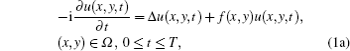where u(x,y,t) is the wave function and f(x,y) is the potential function. The Schrödinger equation has the following initial and boundary conditions: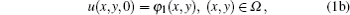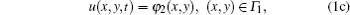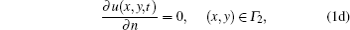where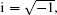T is the time, φ1 (x,y) and φ2 (x,y) are the known functions, Ω = {(x,y)|axb, cyd}, Γ = Γ1Γ2 is the boundary of Ω, and n is the outward normal direction of the boundary Γ2.

By taking variation and the subsection integration of Eq. (1a), the weak form of the unsteady Schrödinger equation is obtained as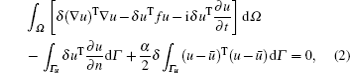where α is the penalty factor.

2.2. IMLS shape function

In the IMLS approximation, we define the following local approximation: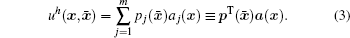Define a functional as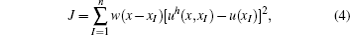where w(xxI) are weight functions, xI are the nodes in influenced domain of x. Equation (4) can be rewritten in the vector form as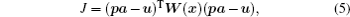where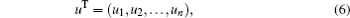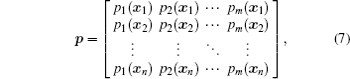and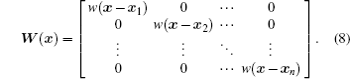By solving the extremum of J, we can find the coefficients a(x) as follows: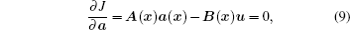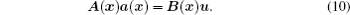If p1 (x),p2 (x), …, pm(x) satisfy the following conditions: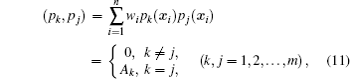then the weighted functions p1(x),p2 (x), …, pm (x) are called an orthogonal function set. The orthogonal function set p = (pi) can be obtained by using Schmidt method as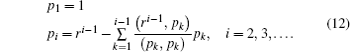Equation (10) can be rewritten as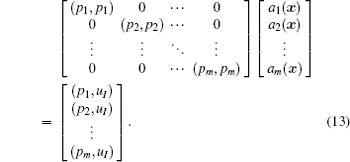The coefficients ai(x) can be obtained as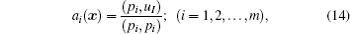i.e.,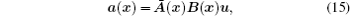where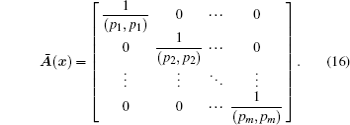From Eq. (15), uh(x) can be written as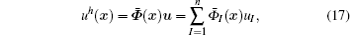where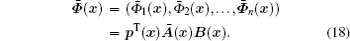By differentiate Eq. (18), we will obtain the derivatives of shape function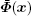as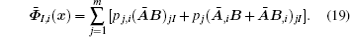The cubic spline weight function is chosen as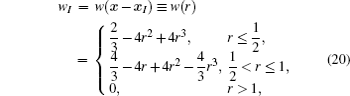where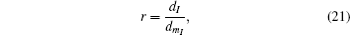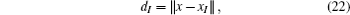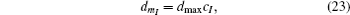and dmax is a scaling parameter, cI is the distance and chosen so that matrix M(x) is not singular. The first and second order derivatives of the weight functions can be easily obtained by chain rule as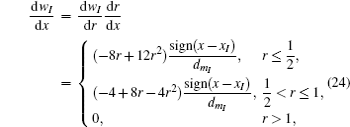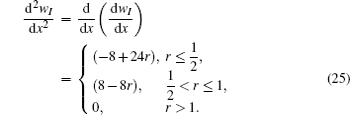2.3. Discretization procedure

From Eq. (17), we obtain the approximation of trial function as follows: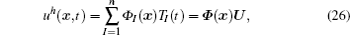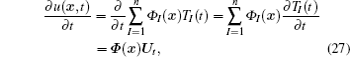where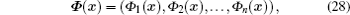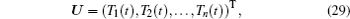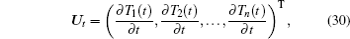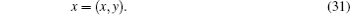Substituting Eqs. (26)–(31) into Eq. (2), we obtain the vector form as follows: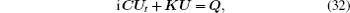where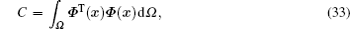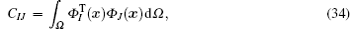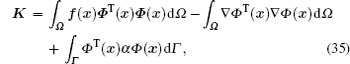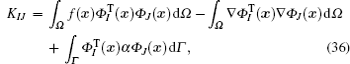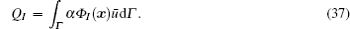Since U and Q are complex numbers, we let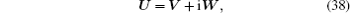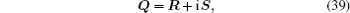where V and W are the real and imaginary part of U; R and S are the real and imaginary parts of Q.

Substituting Eqs. (38) and (39) into Eq. (32), we obtain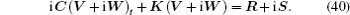Separate the real part from the imaginary part of Eq. (40), we derive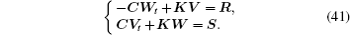By using the center difference method, we make the discretization of Eq. (41) as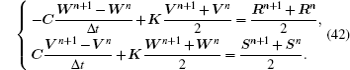Equation (42) is equivalent to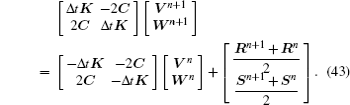The numerical results of 2D Schrödinger equation by the IEFG method can be obtained through solving Eq. (43).

3. Numerical examples

In this section, three numerical examples are demonstrated to verify the efficiency and accuracy of the IEFG method. The implementation of the IEFG method is also presented. In all numerical examples, cubic spline function is chosen as a weight function, and the linear basis function is used.

3.1. Example 1

Consider Eq. (1) on Ω = [0,1] × [0,1] with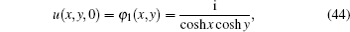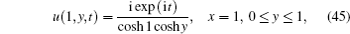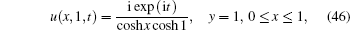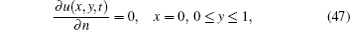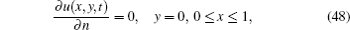and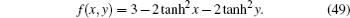The analytical solution is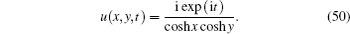The IEFG method is used to solve the above equation with penalty factor α = 105 and dmax = 2.3 in time steps of Δt = 0.01. Figure 1 shows the numerical and solutions for real part of u(x,y,t) at t = 0.5, 1, 2, 3, 4, and 6, respectively. Figure 2 describes the numerical solution and exact solution for imaginary part of u(x,y,t) when t = 0.5, 1, 2, 3, 4, and 6, respectively. In Figs. 3, 4, and 5, the surfaces of real part and imaginary part of u(x,y,t) are plotted when t = 3, 4, and 6, respectively. Table 1 presents the relative errors for the real part and imaginary part of the solution and the CPU time at t = 2. As can seen from Table 1, the smaller time step is chosen, the smaller relative error is obtained. Table 2 shows the relationship between the relative error and number of nodes for the IEFG method at time t = 1. Tables 1 and 2 show that the error norm decreases with the increase of the number of nodes and decrease of time step.

 Figure Option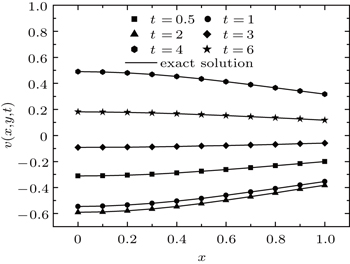Fig. 1. Real parts of exact solution and numerical solution of u(x,y,t).
 Figure Option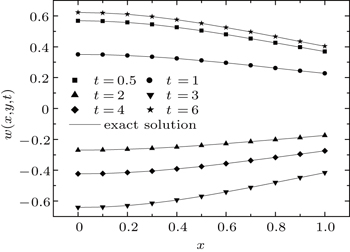Fig. 2. Imaginary parts of exact solution and numerical solution of u(x,y,t).
 Figure Option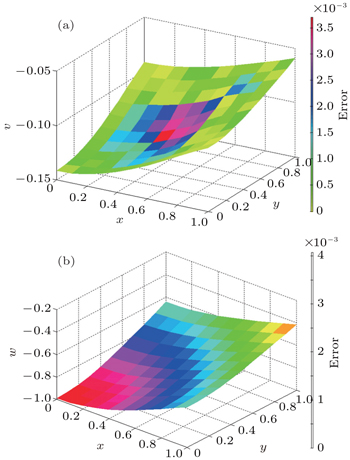Fig. 3. Surfaces of (a) real part and (b) imaginary part of u(x,y,t) at time t = 3.
 Figure Option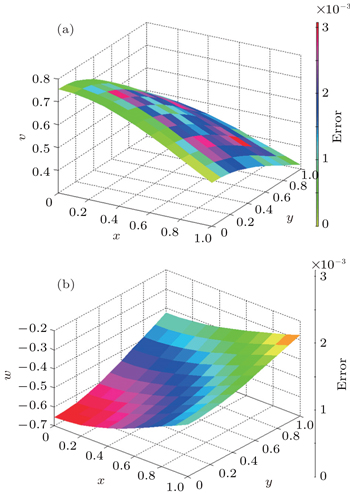Fig. 4. Surfaces of (a) real part and (b) imaginary part of u(x,y,t) at time t = 4.
 Figure Option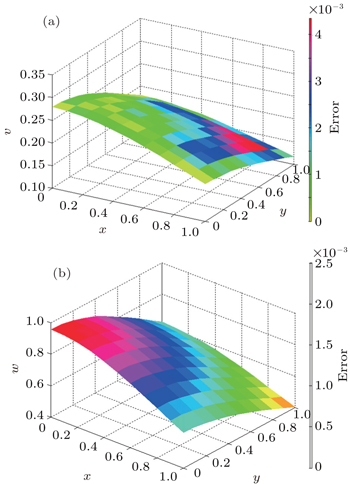Fig. 5. Surfaces of (a) real part and (b) imaginary part of u(x,y,t) at time t = 6.Table 1.

Relative errors and CPU times for the IEFG method at the time t = 2.

.Table 2.

Dependences of relative error on node number for the IEFG method at t = 1.

.
3.2. Example 2

Consider the case f(x,y) = 0 in Eq. (1) on Ω = [0,1] × [0,1] with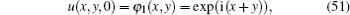which derives the analytical solution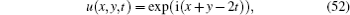and the Dirichlet boundary conditions can be obtained from Eq. (52).

The IEFG method is used with penalty factor α = 105 and dmax = 2.3 in time steps of Δt = 0.01.

Figure 6 shows the numerical solution and analytical solution for real part of u(x,y,t) at time t = 0.5, 1, 2, 3, 4, and 6, respectively. Figure 7 shows the numerical solution and exact solution for imaginary part of u(x,y,t) at t = 0.5, 1, 2, 3, 4, and 6, respectively. In Figs. 810, the surfaces of real part and imaginary part of u(x,y,t) are plotted at t = 1, 3, and 6, respectively.

 Figure Option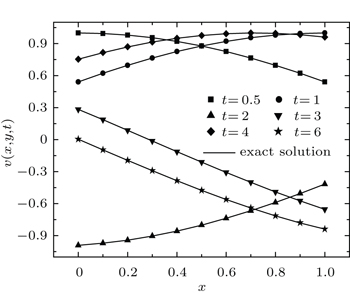Fig. 6. Real parts of analytical solution and numerical solution of u(x,y,t).
 Figure Option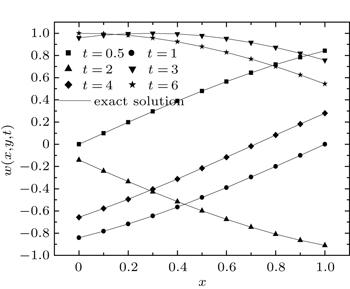Fig. 7. Imiginary parts of analytical solution and numerical solution of u(x,y,t).
 Figure Option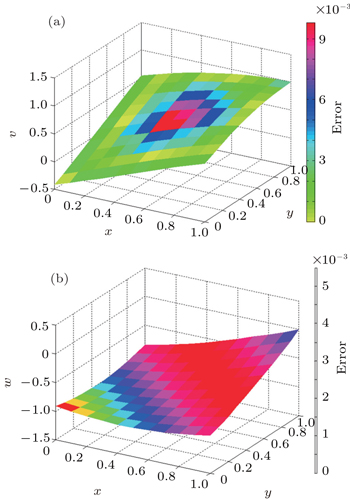Fig. 8. Surfaces of (a) real part and (b) imaginary part of u(x,y,t) at time t = 1.
 Figure Option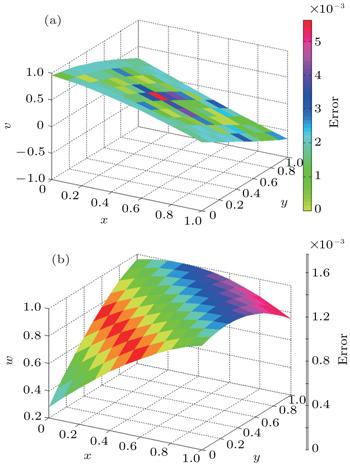Fig. 9. Surfaces of (a) real part and (b) imaginary part of u(x,y,t) at time t = 3.
 Figure Option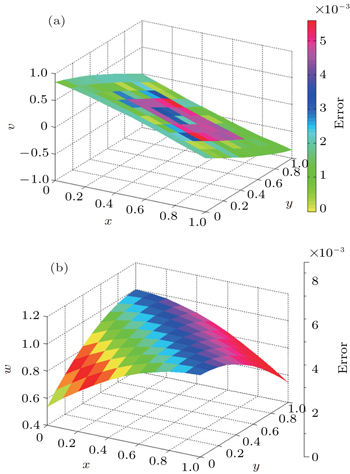Fig. 10. Surfaces of (a) real part and (b) imaginary part of u(x,y.t) at time t = 6.
3.3. Example 3

Consider Eq. (1) on Ω = [0, 1] × [0, 1] with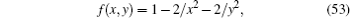and the analytical solution is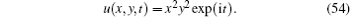The initial and boundary conditions are easily derived from Eq. (54) as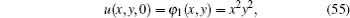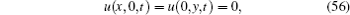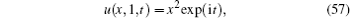The IEFG method is used with penalty factor α = 105 and dmax = 2.3 in time steps of Δt = 0.01. Figure 11 shows the numerical solution and analytical solution for real part of u(x,y,t) when t = 0.5, 1, 2, 3, 4, and 6, respectively. Figure 12 describes the numerical solution and exact solution for imaginary part of u(x,y,t) when t = 0.5, 1, 2, 3, 4, and 6, respectively. The surfaces of real and imaginary part of u(x,y,t) are plotted at t = 1, 3, and 6, respectively, in Figs. 1315.

 Figure Option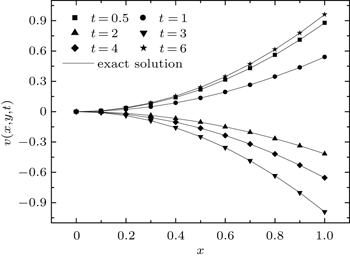Fig. 11. Real parts of analytical solution and numerical solution of u(x,y,t).
 Figure Option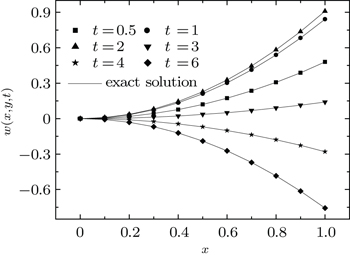Fig. 12. Imaginary parts of analytical solution and numerical solution of u(x,y,t).
 Figure Option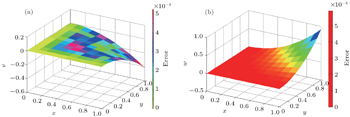Fig. 13. Surfaces of (a) real part and (b) imaginary part of u(x,y,t) at time t = 6.
 Figure Option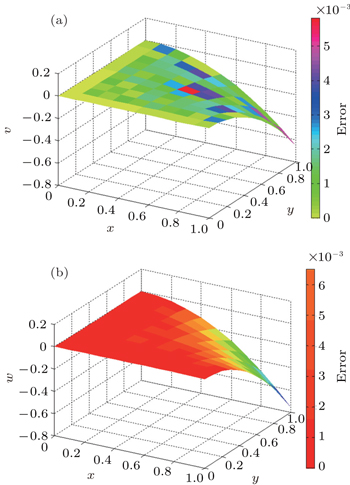Fig. 14. Surfaces of (a) real part and (b) imaginary part of u(x,y,t) at time t = 4.
 Figure Option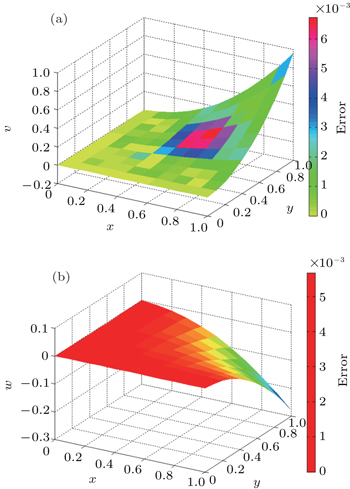Fig. 15. Surfaces of (a) real part and (b) imaginary part of u(x,y,t) at time t = 6.

From these figures, it is evident that the present numerical results obtained by the IEFG method are in excellent agreement with the analytical solutions.

4. Conclusions

The IEFG approach to solving 2D Schrödinger equation is developed and numerically implemented in the present work. The IMLS approximation is used to approximate the 2D trial function. By using the variation method, the final algebraic equation system is obtained due to energy functional. In the IMLS approximation, the basis function is chosen as an orthogonal function system, and the resulting final equation system is no more ill-conditioned due to involving no inverse matrix. It is demonstrated that the IMLS technique has a higher efficiency and precision than the traditional MLS approximation. Owing to its simple implementation, the IEFG method has the potential to replace the difference method and the finite element method for solving 2D Schrödinger equation and any other nonlinear partial differential equation.

Reference
 1 Kivshar YAgrawal G P2003Optical Solitons: From Fibers to Photonic CrystalsNew YorkAcademic Press 2 Deng Y XTu C HLu F Y2009Acta Phys. Sin.583173(in Chinses) 3 Zhao LSui ZZhu Q HZhang YZuo Y L2009Acta Phys. Sin.584731(in Chinses) 4 Hasegawa A1989Optical Solitons in FibersBerlinSpringer-Verlag 5 Dodd R KEilbeck J CGibbon J DMorris H C1982Solitons and Nonlinear Wave EquationsNew YorkAcademic Press 6 Pitaevskii L PStringari S2003Bose–Einstein CondensationOxfordOxford University Press 7 Scott A1999Nonlinear Science: Emergence and Dynamics of Coherent Structures Vol. 1OxfordOxford University Press 8 Arnold A 1998 VLSI Design 6 313 9 Levy M2000Institution of Electrical Engineers London 10 Tappert F D1977Lecture Notes in PhysicsBerlinSpringer 11 Kopylov Y VPopov A VVinogradov A V 1995 Opt. Commun. 118 619 12 Huang WXu CChu S TChaudhuri S K 1992 J. Lightwave Technology 10 295 13 Subasi M 2002 Numerical Methods for Partial Differential Equations 18 752 14 Antoine XBesse CMouysett V 2004 Math. Comput. 73 1779 15 Kalita J CChhabra PKumar S 2006 J. Comput. Appl. Math. 197 141 16 Dehghan MShokri A 2007 Comput. Math. Appl. 54 136 17 Dehghan M 2006 Math. Comput. Simul. 71 16 18 Dehghan MMirzaei D 2008 Engineering Analysis with Boundary Elements 32 747 19 Ang W TAng K C 2004 Numerical Methods for Partial Differen-tial Equations 20 843 20 Belytschko TKrongauz YOrgan DFleming MKrysl P 1996 Comput. Method Appl. Mech. Eng. 139 3 21 Cheng Y MLi J H 2006 Sci. China Ser. G Phys. Mech. Astron. 49 46 22 Cheng R JCheng Y M 2008 Appl. Numer. Math. 58 884 23 Peng M JCheng Y M 2009 Engineering Analysis with Boundary Elements 33 77 24 Li D MBai F NCheng Y MLiew K M 2012 Comput. Methods Appl. Mech. Eng. 233�?36 1 25 Lancaster PSalkauskas K 1981 Math. Comput. 37 141 26 Cheng Y MChen M J 2003 Acta Mech. Sin. 35 181 27 Cheng Y MPeng M J 2005 Sci. China-Ser. G Phys. Mech. Astron. 48 641 28 Liew K MCheng Y MKitipornchai S 2006 Int. J. Numer. Methods Eng. 65 1310 29 Kitipornchai SLiew K MCheng Y M 2005 Comput. Mech. 36 13 30 Liew K MCheng Y MKitipornchai S 2005 Int. J. Numer. Methods Eng. 64 1610 31 Zhang ZLiew K MCheng Y M 2008 Engineering Analysis with Boundary Elements 32 100 32 Zhang ZLiew K MCheng Y MLi Y Y 2008 Engineering Anal-ysis with Boundary Elements 32 241 33 Zhang ZWang J FCheng Y MLiew K M 2013 Sci. China-Phys. Mech. Astron. 56 1568 34 Zhang ZCheng Y MLiew K M 2013 Engineering Analysis withBoundary Elements 37 1576 35 Ren H PCheng Y MZhang W 2009 Chin. Phys. B 18 4065 36 Ren H PCheng Y MZhang W 2010 Sci. China-Ser. G Phys. Mech. Astron. 53 758 37 Ren H PCheng Y M 2011 Int. J. Appl. Mech. 3 735 38 Ren H PCheng Y M 2012 Engineering Analysis with Boundary Elements 36 873 39 Sun F XWang J FCheng Y MHuang A X 2015 Appl. Numer. Math. 98 79 40 Cheng R JLiew K M 2012 Engineering Analysis with Boundary Elements 36 203 41 Cheng R JLiew K M 2009 Comput. Mech. 45 1 42 Cheng R JLiew K M 2012 Engineering Analysis with Boundary Elements 36 1322 43 Cheng R JLiew K M 2012 Comput. Method Appl. Mech. Eng. 245-246 132 44 Wang J FSun F XCheng Y M 2012 Chin. Phys. B 21 090204 45 Wang J FWang J FSun F XCheng Y M 2013 Int. J. Comput. Methods 10 1350043 46 Sun F XWang J FCheng Y MHuang A X 2015 Appl. Numer. Math. 98 79 47 Chen LCheng Y M2010Sci. China-Ser. G Phys. Mech. Astron.53954 48 Chen LCheng Y M 2010 Chin. Phys. B 19 090204 49 Chen LCheng Y MMa H P 2015 Comput. Mech. 55 591 50 Peng M JLiu PCheng Y M 2009 Int. J. Appl. Mech. 1 367 51 Peng M JLi D MCheng Y M 2011 Engineering Structure 33 127 52 Li D MPeng M JCheng Y M 2011 Sci. China-Ser. G Phys. Mech. Astron. 41 1003 53 Cheng Y MLi R XPeng M J 2012 Chin. Phys. B 21 090205 54 Bai F NLi D MWang J FCheng Y M 2012 Chin. Phys. B 21 020204 55 Cheng Y MLiu CBai F NPeng M J 2015 Chin. Phys. B 24 100202 56 Deng Y JLiu CPeng M JCheng Y M2015Int. J. Appl. Mech.71550017